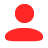Abaqus域级并行

Abaqus将划分好的拓扑域均匀地分配给每个线程，所有域内单独进行计算，每一个时间增量内，有共同边界的域之间会进行通信，合并数据。

Domain如何影响计算结果？

1个拓扑域运行（左）- 2个拓扑域运行（右）

a=F1+F2+F3+F4= -0.06

1个拓扑域运行

a=((F1 + F2) + F3) + F4

a=((.016E2 + .101E2) -.015E2) - .102E2= 0

2个拓扑域运行

a=(F1 + F3) + (F2 + F4)

a=(.157E1 - .153E1) + (.101E2 - .102E2)=.004E1 - .001E2

a=.004E1 - .010E1 = -0.006E1= -0.06

``*IMPERFECTION, FILE=tube_buckle, STEP=1 1, 2.0E-5 2, 0.8E-5 3, 0.4E-5 4, 0.18E-5 5, 0.16E-5 6, 0.10E-5 7, 0.10E-5 8, 0.08E-5 9, 0.02E-5 10, 0.02E-5``